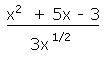## Differentiation

Differentiation allows us to find rates of change. For example, it allows us to find the rate of change of velocity with respect to time (which is acceleration). It also allows us to find the rate of change of x with respect to y, which on a graph of y against x is the gradient of the curve. There are a number of simple rules which can be used to allow us to differentiate many functions easily.

If y = some function of x (in other words if y is equal to an expression containing numbers and x's), then the derivative of y (with respect to x) is written dy/dx, pronounced "dee y by dee x" .

Differentiating x to the power of something

1) If y = xn, dy/dx = nxn-1

2) If y = kxn, dy/dx = nkxn-1(where k is a constant- in other words a number)

Therefore to differentiate x to the power of something you bring the power down to in front of the x, and then reduce the power by one.

Examples

If y = x4, dy/dx = 4x3
If y = 2x4, dy/dx = 8x3
If y = x5 + 2x-3, dy/dx = 5x4 - 6x-4

Example

Find the derivative of:This looks hard, but it isn't. The trick is to simplify the expression first: do the division (divide each term on the numerator by 3x½. We get:
(1/3)x3/2 + (5/3)x½ - x (using the laws of indices).

So differentiating term by term: ½ x½ + (5/6)x + ½x-3/2.

Notation

There are a number of ways of writing the derivative. They are all essentially the same:

(1) If y = x2, dy/dx = 2x
This means that if y = x2, the derivative of y, with respect to x is 2x.

(2) d (x2) = 2x
dx
This says that the derivative of x2 with respect to x is 2x.

(3) If f(x) = x2, f'(x) = 2x
This says that is f(x) = x2, the derivative of f(x) is 2x.

Finding the Gradient of a Curve

A formula for the gradient of a curve can be found by differentiating the equation of the curve.

Example

What is the gradient of the curve y = 2x3 at the point (3,54)?
dy/dx = 6x2
When x = 3, dy/dx = 6× 9 = 54